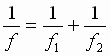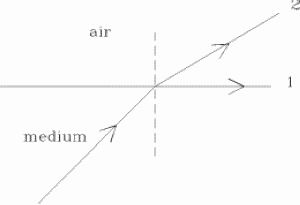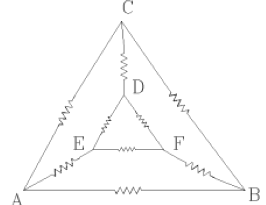PHYSICS-2004

 Time: ONE Hour Max. Marks: 60
• Answers must be written either in English or the medium of instruction of the candidate in high school.

• Answer all the questions in the booklets provided for the purpose.

• There will be no negative marking.

• The relevant working in arriving at an answer has to be shown wherever required.

• Use of calculators or graph papers is not permitted.

• All questions carry equal marks

1. A car traveling at a certain speed v1 overtakes another car traveling at a slower speed v2 at A. An hour later the faster car reverses its direction, changes it’s speed to v3 and after some time meets the slower car now coming in the opposite direction at position B. The distance between the positions A and B is 10 km. Find the speed of the slower car if v1 - v2 = v3 + v2. Find the value of v2.

2. Two lenses of focal lengths f1 and f2 kept in contact can be replaced by a single lens of focal length f. This focal length is given by the following formulaIn using this formula the focal length of a convex lens is taken as positive and the focal length of a concave lens is taken as negative. Use this formula to solve the following problem.
A point object is placed 36 cm away from a convex lens and its image is formed on a screen placed 180 cm away from the lens on the other side. If now a second lens is placed in contact with the first lens, the screen has to be shifted by 90 cm towards the lenses in order to obtain a clear image on it. What is focal length of the second lens? The screen is fixed at this position now and the focal length of the second lens is doubled. Find the distance through which the object has to be shifted to obtain a clear image on the screen.

3. Three conducting wires of equal radii having lengths in the ratio 1:2:3 have their resistivities in the ratio 2:3:4. These wires are connected end to end. When a certain potential difference is applied between the free ends of the combination, potential difference between the ends of middle conductor is found to be 60 V. Find the potential differences between the ends of the other conductors. If the rate of heat production from the first conductor is 4 W, find the rate of heat production from the other two.

4. Two cubes made of same material have some hollow space in them and are floating in a liquid of density 2 g/cm3. One cube is just floating while the other has three fourths of its volume below water. If the cavities in the cubes have their volumes in the ratio 1:2 find the density of the material of the cube.

5. A long horizontal conductor carrying a current is oriented along east west line. An electron located above the conductor and moving eastward experiences a force towards the conductor. Another electron located vertically below the conductor has its velocity directed towards the conductor. Find the direction of force on this electron.

 A certain transparent slab exhibits an interesting property. Its refractive index has two different values for a given wavelength. A ray of light is passing from this medium in to air and is incident at a certain angle at its interface with air. The refracted ray 1 for which the refractive index with respect to air is Ö 2 grazes the surface of separation and the other emerges in to air making an angle of 30° with the first refracted ray. Find the angle between the incident and the second refracted rayIf two charged conducting spheres are connected by a wire charge flows from one to the other till the charges on them are in the ratio of their radii. Two small spheres having radii in the ratio 2:1 have charges of ¯2Q and Q on them and are sufficiently far apart to be treated as point charges. They exert force of F on each other. A conducting wire now connects them. Find the force between them after the charges get redistributed on them.
 All resistances shown in the figure are equal. Equivalent resistance between A and B when resistance between C and D is removed is R. The removed resistance is reconnected and the resistances between the corners B,C and D,E are now removed. What is the equivalent resistance between A and B now.A horizontal force of 10 N acts on a block of mass 5 kg lying on a smooth horizontal surface. The block is initially at rest. This force stops acting after 5 s and the body continues to move. 5 s later a force of 20 N starts acting against the motion of the block till the block stops. What is total distance covered by the block. Two cubes made of same material are placed alongside. When a temperature difference of 100 C° is maintained between their farther faces, heat is found to be conducted at a rate R through them. The cubes are now placed one above the other. If the same temperature difference is maintained between the bottom and top faces find the new rate of heat flow. Also find the temperature difference between the bottom and top faces for the rate of heat flow to be R again. (Heat flow is analogous to current flow). A hollow tube is 68 m long. When struck at one end an observer at the other end receives two notes, one due to sound traveling in air and the other through the metal. Estimate the time interval between receiving the sound notes. (Speed of sound in air = 340 m/s, Young’s modulus of the material of the tube = 2 × 1011 N/m2 and density of the material = 8 g/cm3). About 0.2 amu of mass disappears in the fission of a single uranium nucleus (mass number 235). Find the energy released in the fission of 4.7 g of uranium. Express this energy in joule.